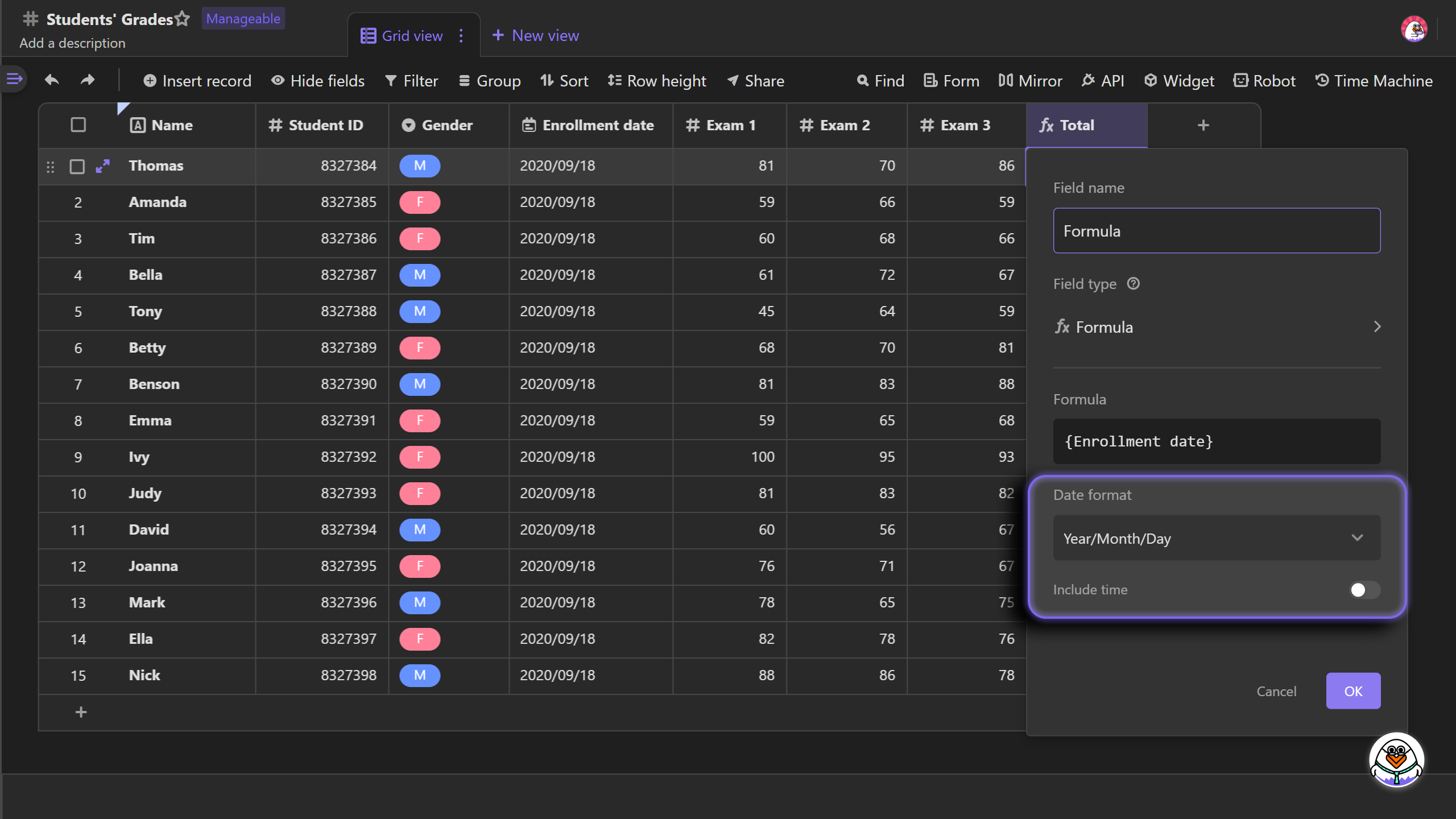# Get to Know Formulas

A formula is an operation expression that is written with a combination of functions, mathematical symbols, and parameters. The input value will be calculated by the formula to get the expected output value.

For example, you want to calculate the total grades of a student's Exam 1, Exam 2, Exam 3. You can use the following formula to do the calculation.

``SUM({Exam 1}, {Exam 2}, {Exam 3})``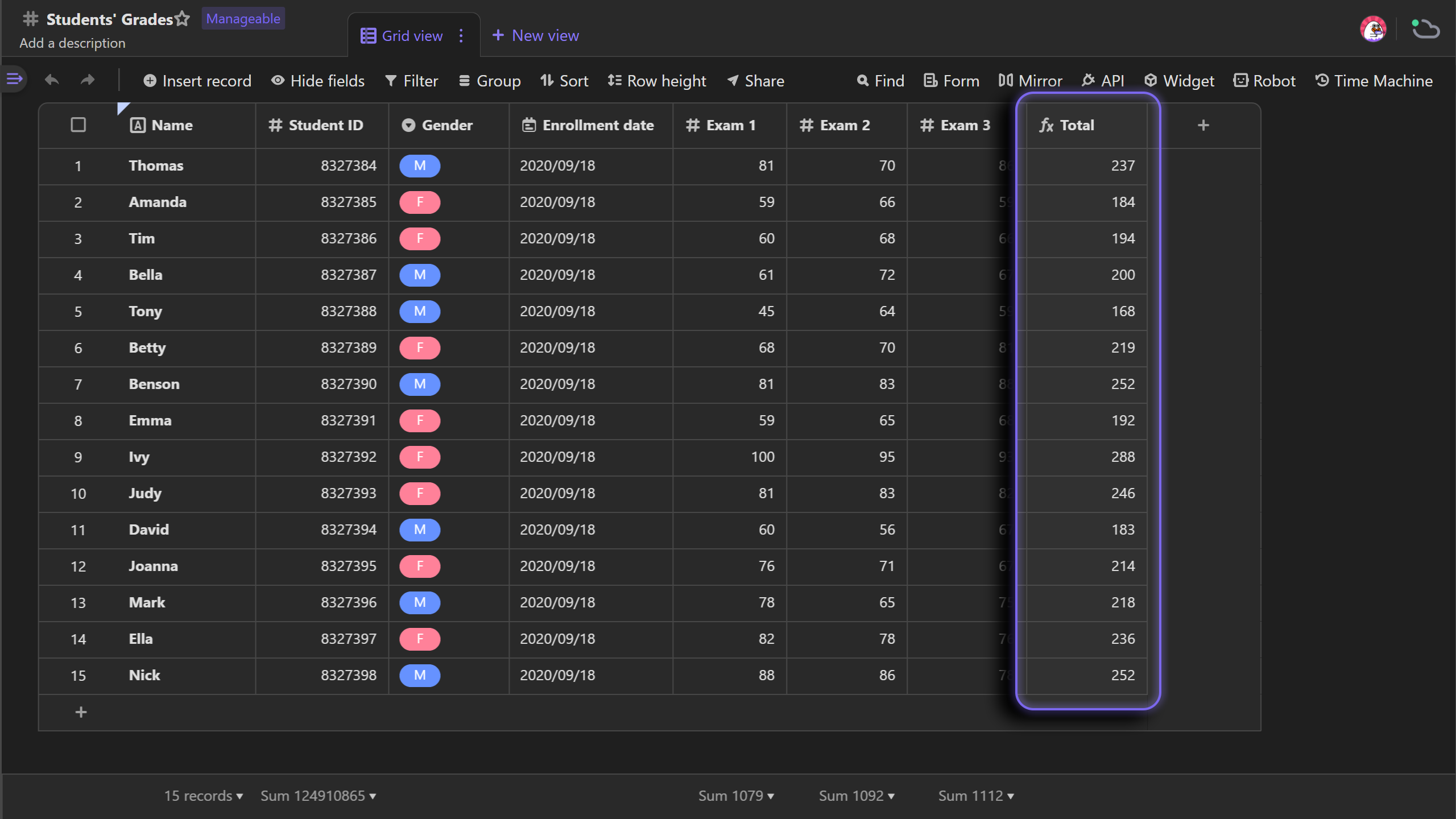## Writing formulas in AITable vs Excel​

Although the primary principles of formula in AITable are similar to those in Excel, there is a small difference:

Formulas in AITable reference the entire fields, rather than a specific cell.

For the example above, to calculate the total grades of a student, you need to reference three separate cells of data in Excel:

Formula：`E2+F2+G2`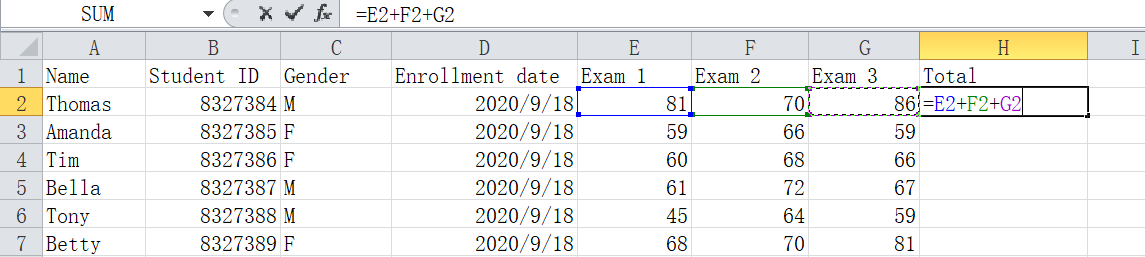In AITable, you only need to reference the fields of "Exam 1", "Exam 2" and "Exam 3":

Formula：`{Exam 1}, {Exam 2}, {Exam 3}`For the example above, the formula in Excel only calculate Thomas' grades, while that in AITable applies to every record in that field, calculating all students' total grades.

## Quick Start​

### Creating a formula​

To create a formula, you need to insert a new field first, and customize the field type to 'Formula'. Then click the box to enter the formula.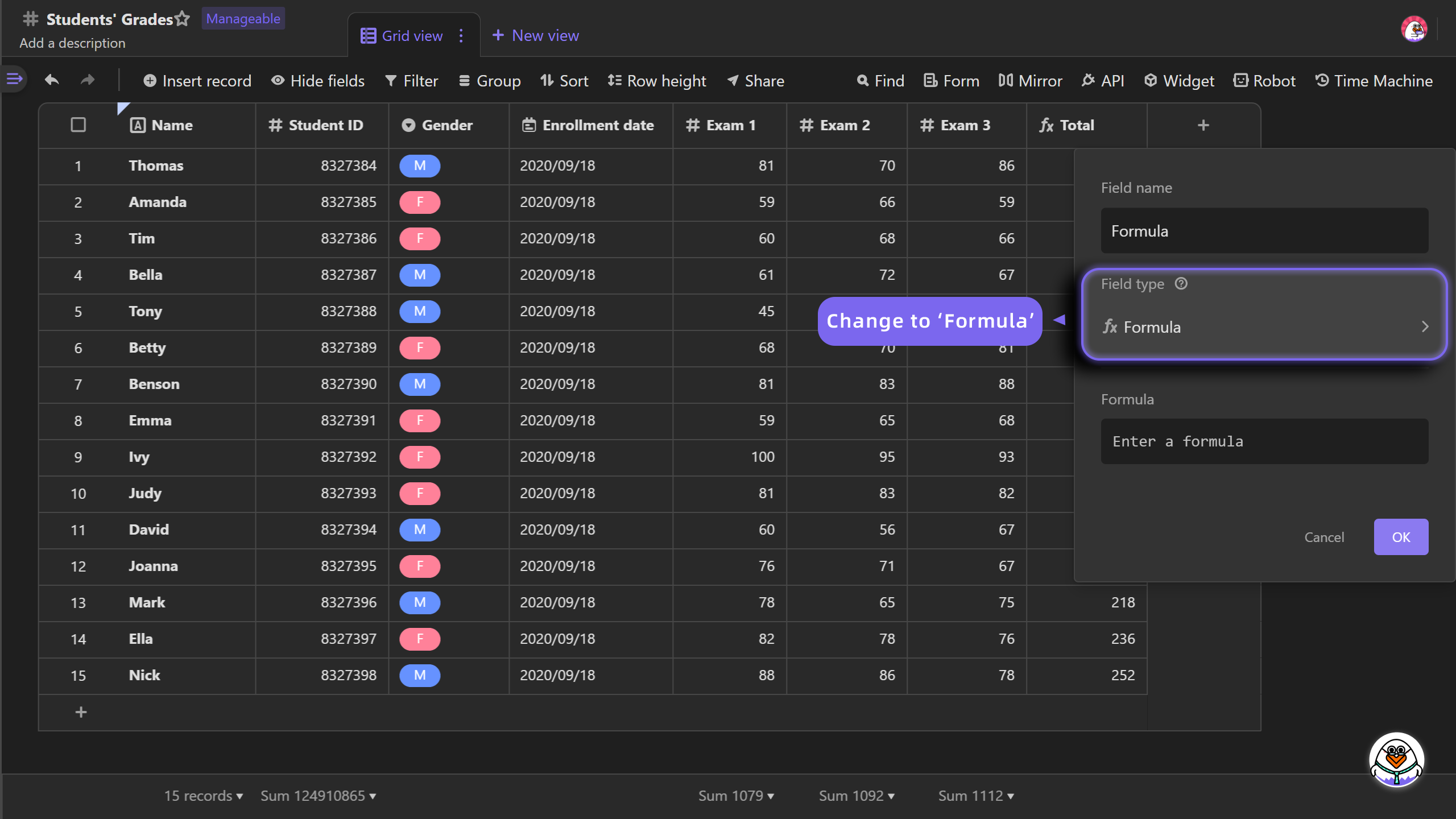### Writing a formula​

Formula dialog consists of three sections. While the head of the box in the dialog is used to write formulas and the left side section of the directory provides references of fields and functions to be picked, the right side section showcase the use of functions.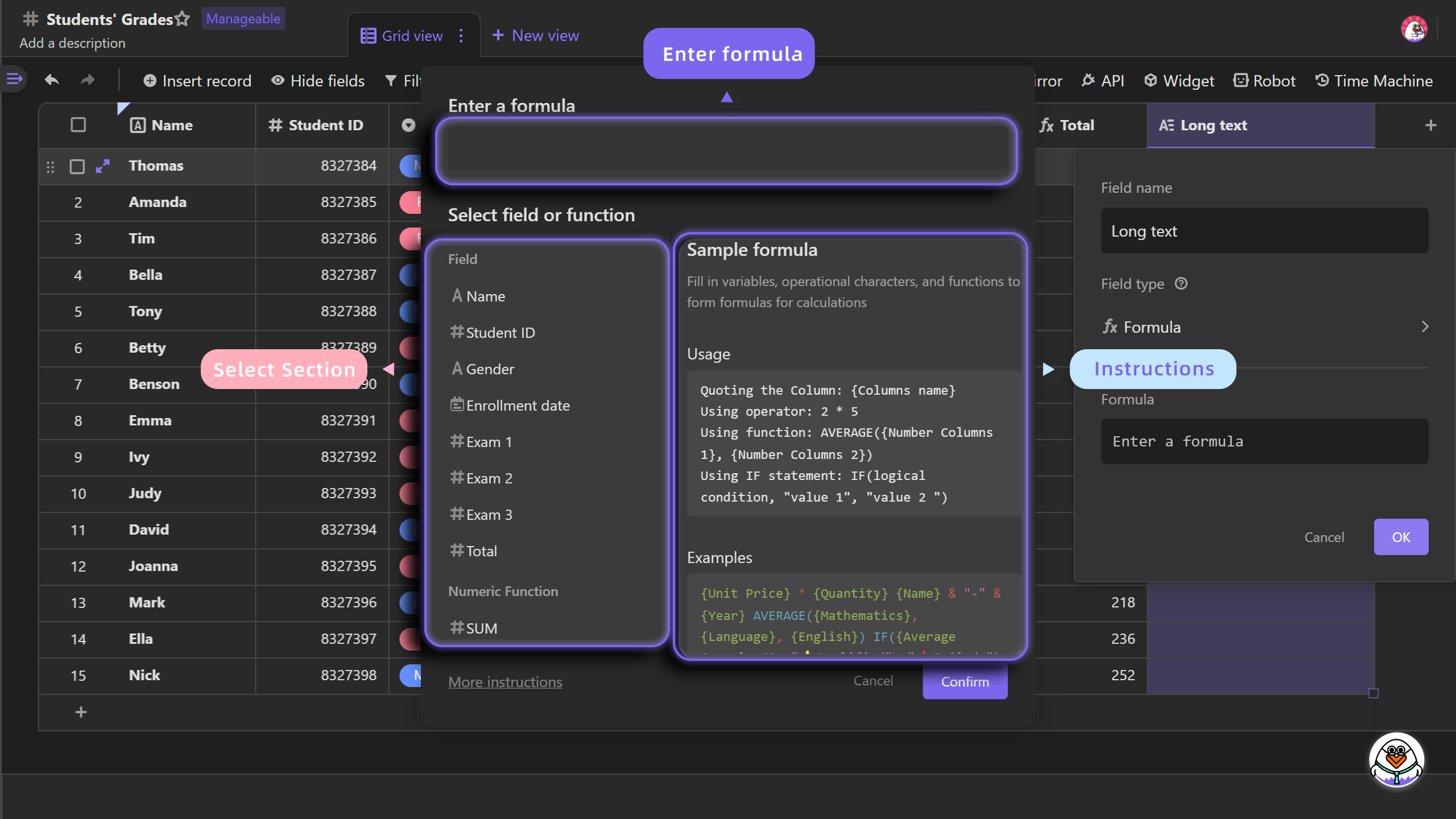Before you start writing a formula, there are some basic principles you need to know.

• Fields: columns in your datasheet, you can reference the entire fields to do calculation.

For example, reference the "Name" field: {name}

• Functions: defined arithmetical formulas for calculating input values to output values.

For example, the summation function：`SUM({Exam 1}, {Exam 2}, {Exam 3})`

• Operators: symbols that can perform logical and arithmetic operations.

For example：+-*/

• Parameters: The data to be entered into the formula to participate in the operation

For example, {Exam 1} and “3”:`({Exam 1} + {Exam 2} + {Exam 3}) / 3`

Let's look at a few more examples to help you understand the formula：

``({Exam 1}+{Exam 2}+{Exam 3})/3=> 86{Name}&”-“&{Student ID}&”-“&{Gerder}=> Thomas-8327384-MMAX({Exam 1}, {Exam 2}, {Exam 3})=> 86IF({Average} > 60, “👍Pass”, “❗Fail”)=>❗Fail``

Let's write a formula now ~

Let's start with some preparation by inserting three Number type fields in the datasheet named 'Exam 1', 'Exam 2' and 'Exam 3'.

Once you fill in the data, create a new field of Formula type. The formula dialog will automatically pop-up after doule clicking on the header of the formula field. Then enter the formula in the box as: `{Exam 1} + {Exam 2} + {Exam 3}`, and click Confirm to exit the dialog. You can see the operation of summation applies to the three fields of 'Exam 1', 'Exam 2' and 'Exam 3'.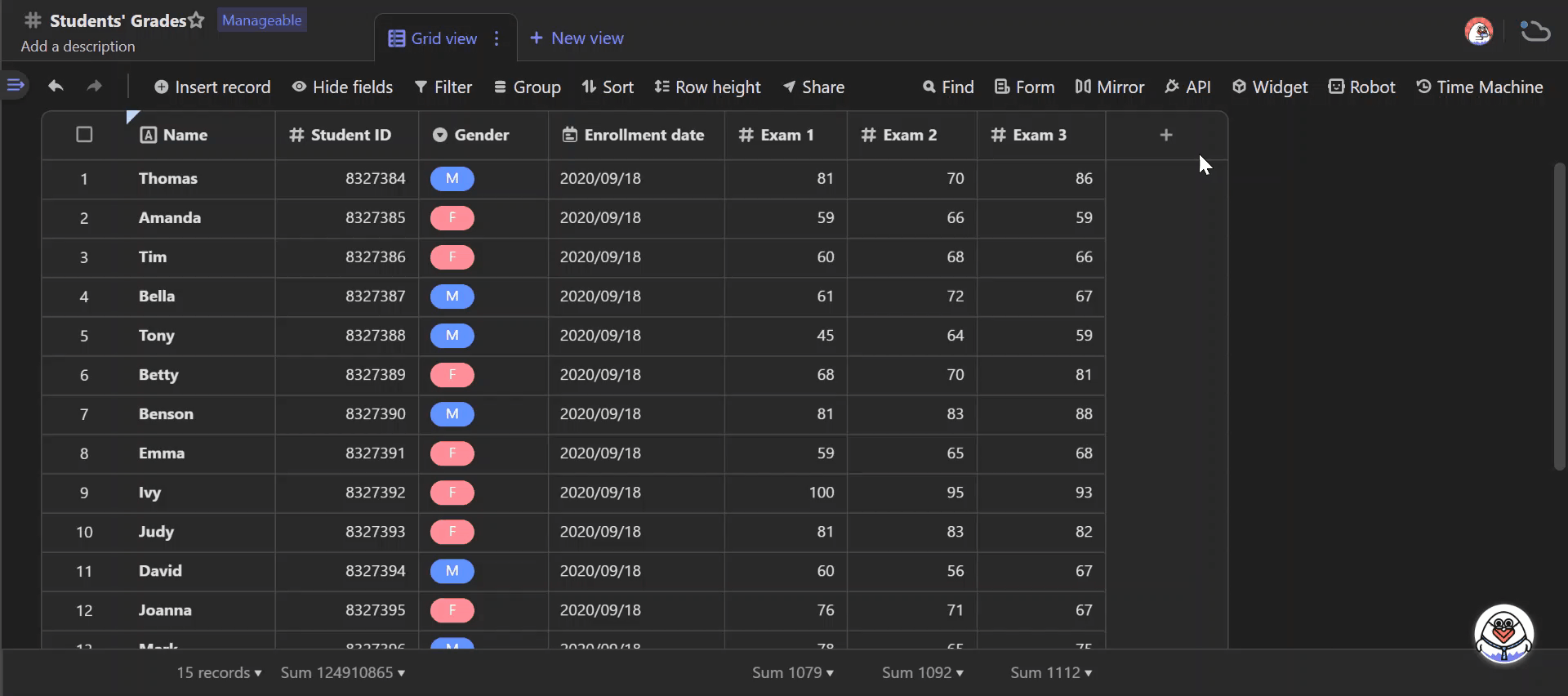### Formatting output​

When the output of a formula is type of Number or Date, we can format the output.

Number

In the example of calculating the total grades of three exams, the type of the formula output is Number, for which you can set decimal places. 1 1.0 1.00 1.000 1.0000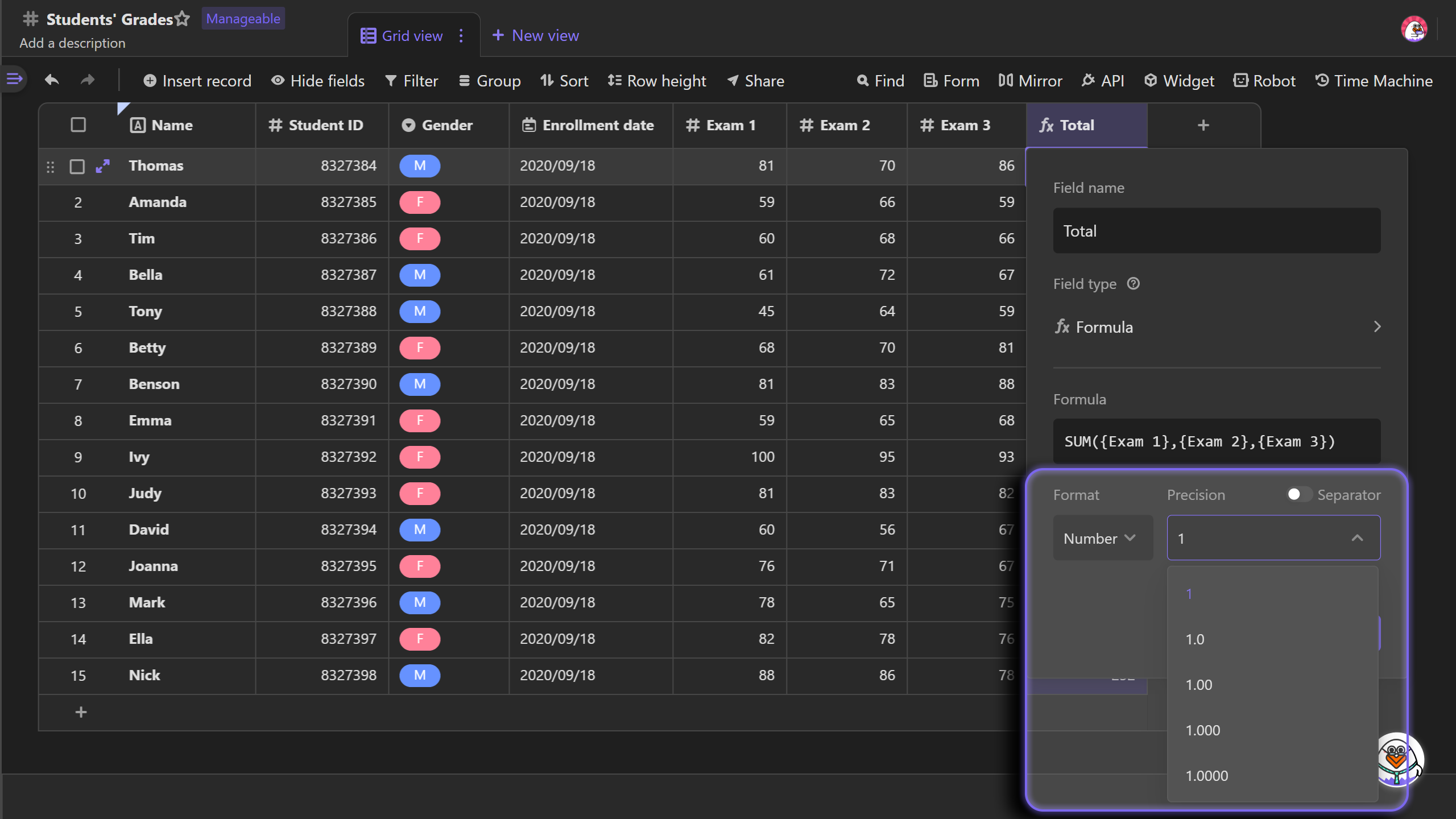Date

If the formula results in a output of Date type, you can choose to display the time or not, and set the date format.

Date format: year/month/day, year-month-day, day/month/year, year-month, month-day, year, month, day